# Introduction to Supervised Learning

## Introduction

Supervised Machine Learning, more commonly known as Supervised Learning, is perhaps the most common sub branch of Machine Learning. Professionals who start their ML journey often begin with Supervised Learning algorithms. The term "supervised" learning refers to the concept that training this sort of algorithm is similar to having a teacher oversee the entire process.

Let's dive into the details without further ado!

## Supervised Learning: How it works

The training data for a supervised learning system will include inputs coupled with the right outputs. This data is then fed as an input to our machine. The algorithm will look for patterns in the data that correspond with the intended outcomes during training. A supervised learning algorithm will take in unknown inputs after training and select which label the new inputs will be categorized based on earlier training data. A supervised learning model's goal is to anticipate the proper label for newly provided input data.

If that was unclear to you, let's take an example to understand the working better.
Let's say our dataset comprises three kinds of shapes: Triangles, Squares, and Hexagons.
We'll train the model by labeling a shape as

• Triangle if it has three sides
• Square if it had four sides
• Hexagon if it has six sides

Now, after training, we use the test set to put our model to the test, and the model's objective is to identify the shape presented to it.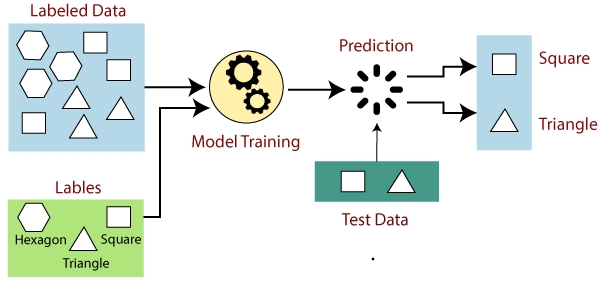source:javatpoint

## Types of Supervised Learning

Supervised learning can be further grouped into two types of subproblems: classification and regression.

### Regression

These algorithms are employed when there is a connection between the input and output variables. Some of the popular regression models include Linear regression, Logistic regression, polynomial regression, etc. We'll be exploring this in more detail later.

### Classification

When the output variable is categorical, classification methods are applied. This identifies particular entities in the dataset and tries to derive inferences about how those items should be labeled or described. We'll explore this in detail in the forthcoming section.

## Regression

In the discipline of machine learning, regression analysis is a crucial concept. In a nutshell, the purpose of a regression model is to create a mathematical equation that describes y as a function of the x variables. Following that, using updated values for the predictor variables (x), this equation may be used to predict the outcome (y).

Let's dive into the types of regression models.

### Types of Regression

Regressions of many forms are used in data science and machine learning. Each kind is essential in various contexts, but at their heart, all regression methods examine the influence of the independent variable on the dependent variables. Here are some of the most significant forms of regression:

• Linear Regression
• Logistic Regression
• Polynomial Regression
• Support Vector Regression
• Decision Tree Regression
• Random Forest Regression
• Ridge Regression
• Lasso Regression

We'll be exploring some of the most important ones later in this post.

## Classification

The Classification method is a Supervised Learning approach that uses training data to identify the category of new observations. An algorithm in Classification learns from a given dataset or observations and then classifies additional observations into one or many classes or categories. A classifier is an algorithm that performs classification on a dataset. Classifiers are broadly divided into two categories:

• Binary classifier: Problems that have only two possible outcomes.
• Multi-class classifier: Problems that have more than two outcomes

There's also one more way classification problems can be divided: based on time spent on training dataset vs. test dataset.

• Lazy learner: Less time for training, More time for prediction.

Example: KNN algorithm.

• Eager Learner: More time in learning, Less time for prediction.

Example: Naive Bayes, Decision trees.

### Types of Classification Algorithms

Classification algorithms can be divided into two main categories.

• Linear Models
• Logistic Regression
• Support Vector Machines
• Non-linear Models
• K-Nearest Neighbours
• Kernel SVM
• Naïve Bayes
• Decision Tree Classification
• Random Forest Classification

## Supervised Learning Algorithms

In supervised machine learning processes, several algorithms and computing approaches are employed. The following are concise descriptions of some of the most popular learning approaches.

Linear regression
It is a statistical regression approach used for predictive analysis. As the name implies,it depicts the linear connection between the independent variable (X-axis) and the dependent variable (Y-axis).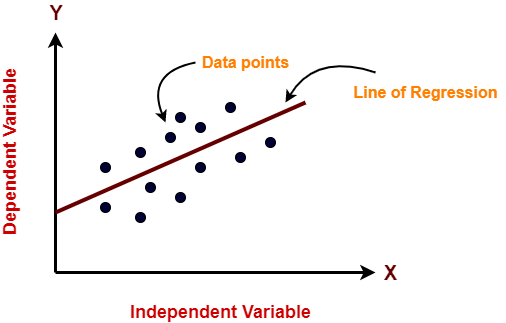source:gatevidyalaya

Logistic regression
It is employed when dependent variables are categorical rather than continuous. While both regression models attempt to identify the links between data inputs, logistic regression is mostly utilized to resolve binary classification problems.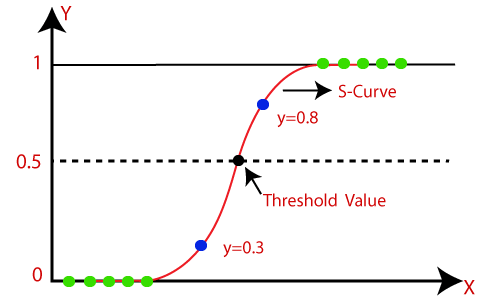source:javatpoint

K-nearest neighbor
The KNN algorithm, also known as the K-nearest neighbor algorithm, is a non-parametric algorithm that classifies data points based on their closeness and relationship to other accessible data.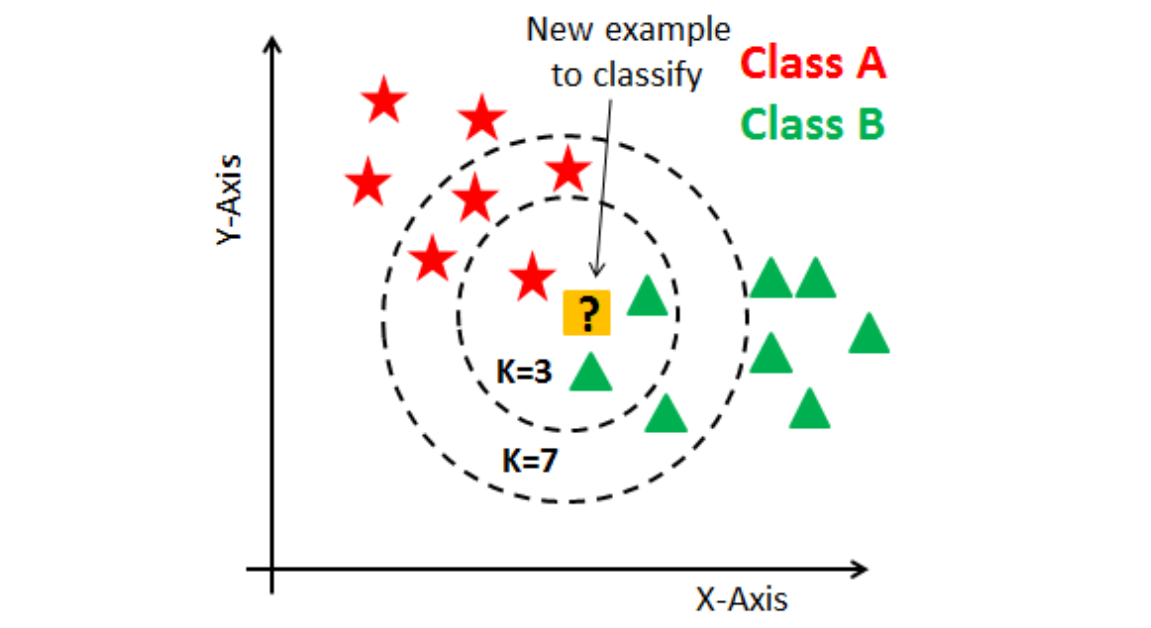source :ai.plainenglish

Support Vector Machine
A support vector machine [SVM] is a common supervised learning model that is used for classification as well as regression problems. However, it is often used for classification problems, creating a hyperplane with the greatest distance between two classes of data points. This hyperplane is known as the decision boundary, and it separates the data point classes.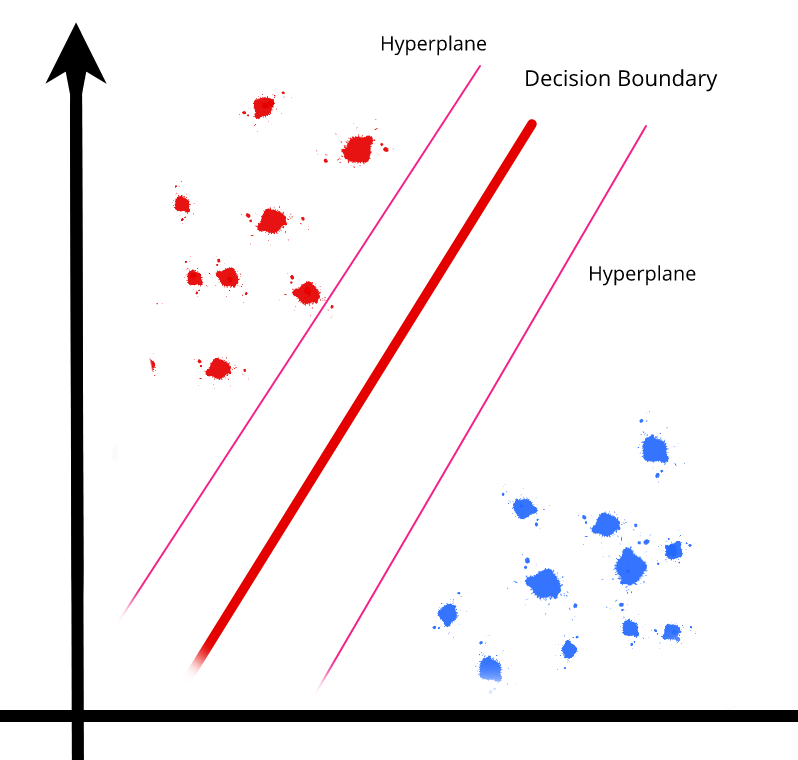source: towardsdatascience

Decision Tree
Decision trees may be used to solve classification as well as regression problems. As the name suggests, It is a tree-like structure in which internal nodes reflect dataset properties, branches represent decision rules, and each leaf node represents the result.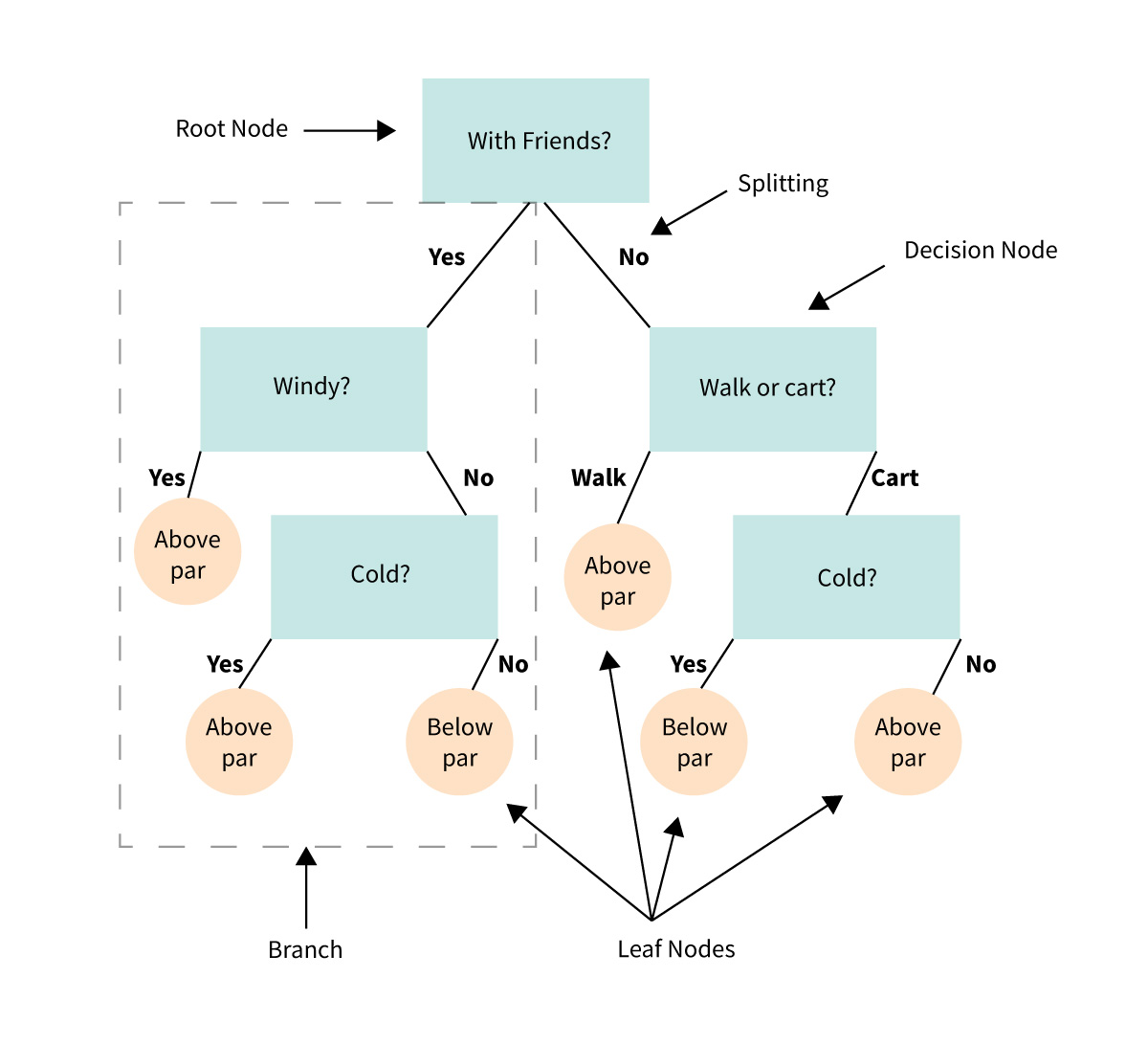source: masterdatascience

Random forests
Random forest is another versatile supervised machine learning technique that may be used for classification and regression. The term "forest" refers to a set of uncorrelated decision trees that are subsequently blended to minimize variation and produce more accurate data predictions.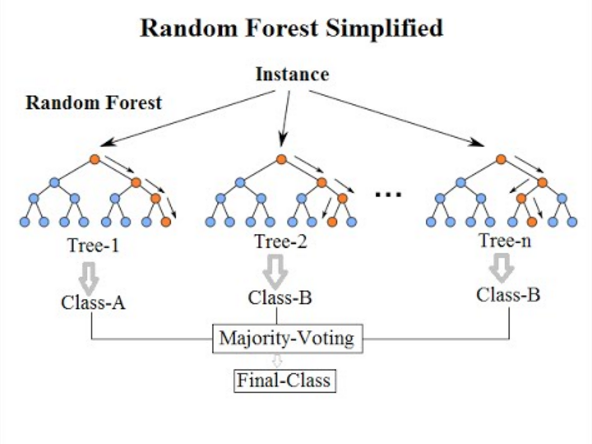source:wikipedia

## Pros and cons of Supervised Learning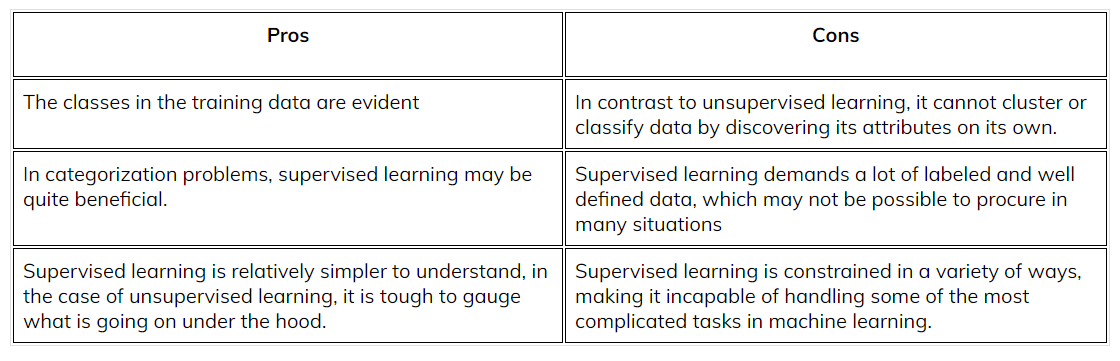## Applications of Supervised Learning

• Analyzing customer sentiment
Organizations can extract and identify significant bits of information from massive amounts of data using supervised machine learning algorithms, including context, emotion, and purpose, with minimal human interaction. This may be pretty helpful in acquiring a better understanding of consumer interactions and improving brand engagement initiatives.
• Spam detection
Another example of a supervised learning model is spam detection. Organizations may train databases to spot patterns or abnormalities in new data using supervised classification algorithms, allowing them to efficiently categorize spam and non-spam correspondences.
• Predictive analytics
Predictive analytics systems that give deep insights into numerous business data points are a common use case for supervised learning models. This enables organizations to forecast certain outcomes depending on a particular output variable, assisting business executives in justifying actions or pivoting for the benefit of the firm.

1. What are some challenges faced in incorporating Supervised Learning?
Preparing and pre-processing data is always a difficulty. Also, incorrect results may be produced by an irrelevant input characteristic present in training data. Unlike unsupervised learning algorithms, supervised learning does not have the ability to cluster or categorize data independently.

2. What are some good practices to keep in mind while working with Supervised learning algorithms?
A pivotal point to keep in mind is the selection of data for the training dataset. Deciding on the appropriate algorithm is also crucial. Finally, verifying the outputs using comparable outputs from human experts or other measures might also help.

3. What is the difference between regression and classification?
The process of determining the relationship between the dependent and independent variables is known as regression whereas classification is the process of identifying a function that aids in categorizing data.

## Key Takeaways

Supervised learning is all about working with labeled data. Supervised learning models may be an effective way to eliminate human categorization efforts and make future predictions based on labeled data. To avoid overfitting data models, however, configuring your machine learning algorithms involves human knowledge and experience. This is often overlooked, but it must be kept in mind.
If you're interested in learning more about ML, check out this course.

Happy learning!